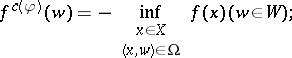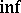# Fenchel-Moreau conjugate function

Given two sets $X$, $W$ and a "coupling" function $\varphi : X \times W \rightarrow \overline { \mathbf{R} }$, the Fenchel–Moreau conjugate to a function $f : X \rightarrow \overline { \mathbf{R} }$ with respect to the coupling function $\varphi$ is the function $f ^ { c \langle \varphi \rangle } : W \rightarrow \overline { \mathbf{R} }$ defined by

\begin{equation} \tag{a1} f ^ { c ( \varphi ) } ( w ) = \operatorname { sup } _ { x \in X } \{ \varphi ( x , w ) - f ( x ) \} ( w \in W ), \end{equation}

with the convention $( + \infty ) - ( + \infty ) = - \infty - ( - \infty ) = - \infty$ [a1]. When $X$ and $W$ are linear spaces in duality, via a bilinear coupling function $\varphi$ (cf. also Linear space; Duality), $f ^ { c ( \varphi )}$ is just the usual Fenchel conjugate $f ^ { * }$ (called also the Young–Fenchel conjugate, or Legendre–Fenchel conjugate; cf. also Legendre transform) of $f$. If $X$ is a locally convex space and $W = X ^ { * },$ the conjugate space of $X$, with the coupling function $\varphi ( x , w ) = w ( x )$, then the second Fenchel conjugate $f ^ { * * } = ( f ^ { * } ) ^ { * }$ of $f$ coincides with the greatest lower semi-continuous minorant of $f$ (Moreau's theorem); this result admits a natural extension to Fenchel–Moreau conjugates $f ^ { c ( \varphi )}$.

Another important particular class of Fenchel–Moreau conjugates is obtained for coupling functions $\varphi : X \times W \rightarrow \overline { \mathbf{R} }$ that take only the values $0$ and $- \infty,$ or, equivalently, the conjugates for which there exists a (unique) subset $\Omega$ of $X \times W$ such that(a2)

these are called conjugates of type Lau or level-set conjugates. While Fenchel conjugates have many applications in convex analysis, conjugates of type Lau are useful for the study of quasi-convex functions (i.e., of functions all of whose level sets are convex) and for duality theory in micro-economics (duality between direct and indirect utility functions).

A useful related concept is the Flachs–Pollatschek conjugate function $f ^ { \Delta ( \varphi ) } : W \rightarrow \overline {\bf R }$, defined by

\begin{equation} \tag{a3} f ^ { \Delta ( \varphi ) } ( w ) = \operatorname { sup } _ { x \in X } \operatorname { min } \{ \varphi ( x , w ) , - f ( x ) \} ( w \in W ), \end{equation}

which has applications in, e.g., optimization theory.

A unified approach is the conjugate function with respect to a binary operation $\odot$ on $\overline{\mathbf{R}}$, assumed completely distributive (cf. also Completely distributive lattice) with respect toin the lattice $( \overline { \mathbf{R} } , \leq )$, defined by

\begin{equation} \tag{a4} f ^ { b ( \varphi ) } ( w ) = \operatorname { sup } _ { x \in X } \{ - [ - \varphi ( x , w ) \odot f ( x ) ] \} ( w \in W ); \end{equation}

in particular, when $\odot = +$ (respectively, $\odot=\max$), $f ^ { b ( \varphi ) }$ is the Fenchel–Moreau (respectively, the Flachs–Pollatschek) conjugate function of $f$.

In another direction, the Fenchel–Moreau conjugate has been generalized to functions with values in extensions $\overline { G }$ of ordered groups $G$, with applications to functions in the extension (by adjoining $- \infty$ and $+ \infty$) of the additive group $( \mathbf{R} , + , \leq )$ and to functions in the extension (by adjoining $0$ and $+ \infty$) of the multiplicative group $( \mathbf{R} _ { + } \backslash \{ 0 \} , \times , \leq )$. More generally, one has also defined the conjugate function of $f : X \rightarrow \overline { G }$ with respect to a binary operation $\odot$ on $\overline { G }$, encompassing the preceding conjugates as particular cases.

One of the main fields of applications of these concepts is optimization theory: When $f$ is the objective function of an optimization problem, a conjugate function is used to define (the objective function of) a "dual" optimization problem.

For more details, see [a2], [a3], [a4].